# Closed Captioned Video: Algebra Nspirations: Data Analysis and Probability

What are the two meanings of statistics? What does it really mean that an event has a 50% probability of occurring? Why are data analysis and probability always taught together? Written and hosted by internationally acclaimed math educator Dr. Monica Neagoy, this video answers these questions and addresses fundamental concepts such as the law of large numbers and the notion of regression analysis. Both engaging investigations are based on true stories and real data, utilize different Nspire applications, and model the seamless connection among various problem representations. Concepts explored: statistics, data analysis, regression analysis.

## Exponential Functions

### Brief Review of Exponents

When a number is raised to a power, exponents are involved. Here are the key components of an exponential expression.

Exponents make it easier to write expressions that would otherwise be cumbersome to write. See how much easier it is to write 310 instead of the expression on the right?Exponents are a different form of writing numbers. With this new form come new properties that are unique to exponents. The next section goes over these properties, also known as the Laws of Exponents.

### Laws of Exponents

The Laws of Exponents are a set of properties that pertain to all exponential expressions. Study the definition below.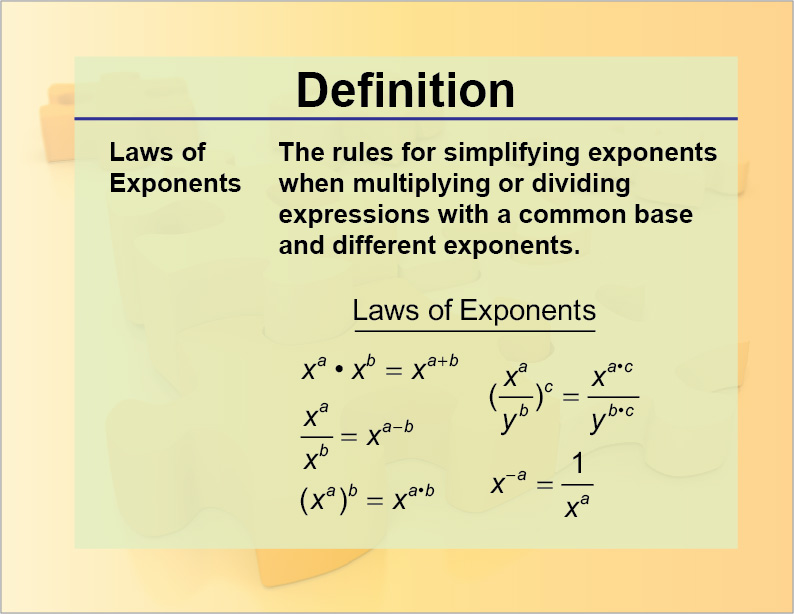To learn more about exponents, click on this link. It is a presentation that goes over the Laws of Exponents in more detail. To see examples that use the Laws of Exponents, click on this link

 Exponential Expressions Example 1 What is the value of x?In order to work with the Laws of Exponents, make sure the terms have the same base. Both 8 and 32 can be written as powers of 2:Rewrite the original expression with these powers of 2:With a common base, you can now solve for x:Example 2 If 4x - 5y = 10, what is the value of this expression?Find a common base for the numerator and denominator, then simplify the expression:Do you notice that the exponent for 2 is the same as the left side of the linear equation? Replace the exponentsExample 3 Rewrite this expression as an exponential expression with a power of 5.First, write the numerical expression as an exponential expression:Next, rewrite 125 as a power of 5:Finally, rewrite the entire expression:### Exponential Functions

An exponential function is of this form:In this function the terms a, b, and c are numbers and x is the independent variable. Here’s one of the simplest exponential functions:This is the graph of the function.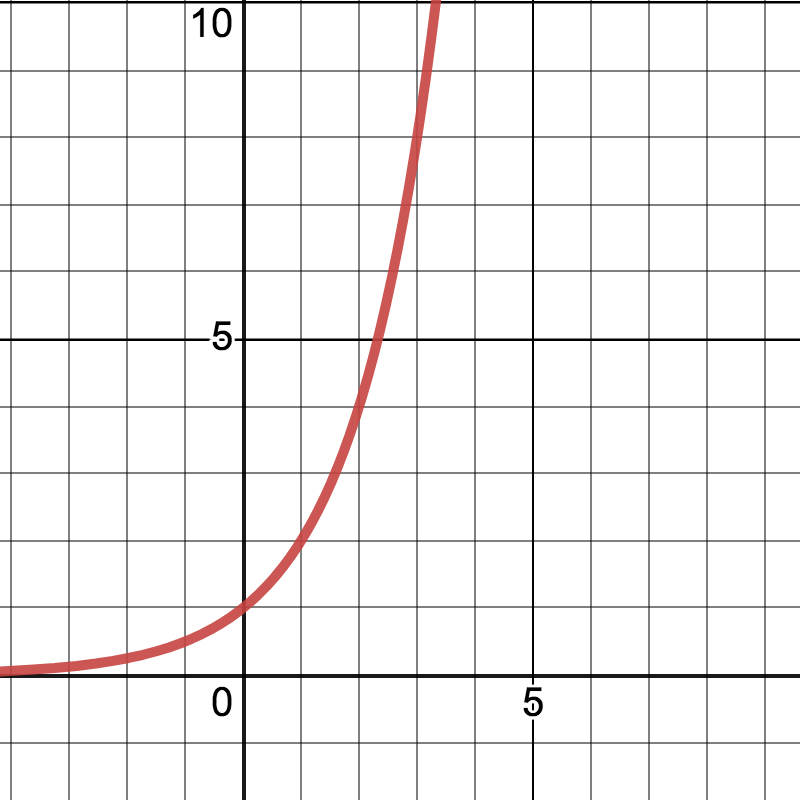As you can see from the graph, the values of f(x) increase dramatically for small increases in input values of x. Here are some other examples of exponential functions.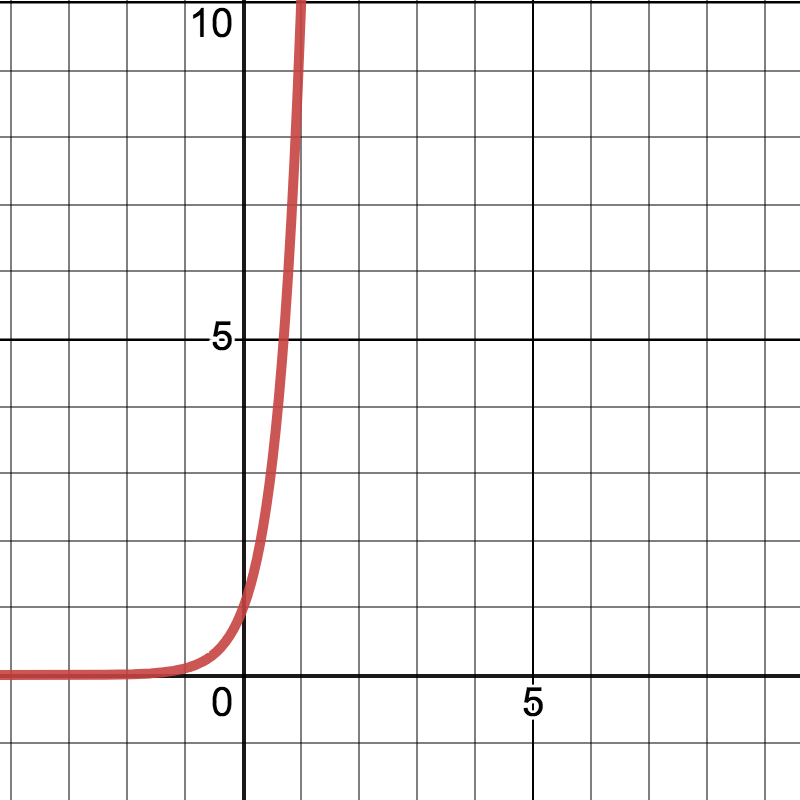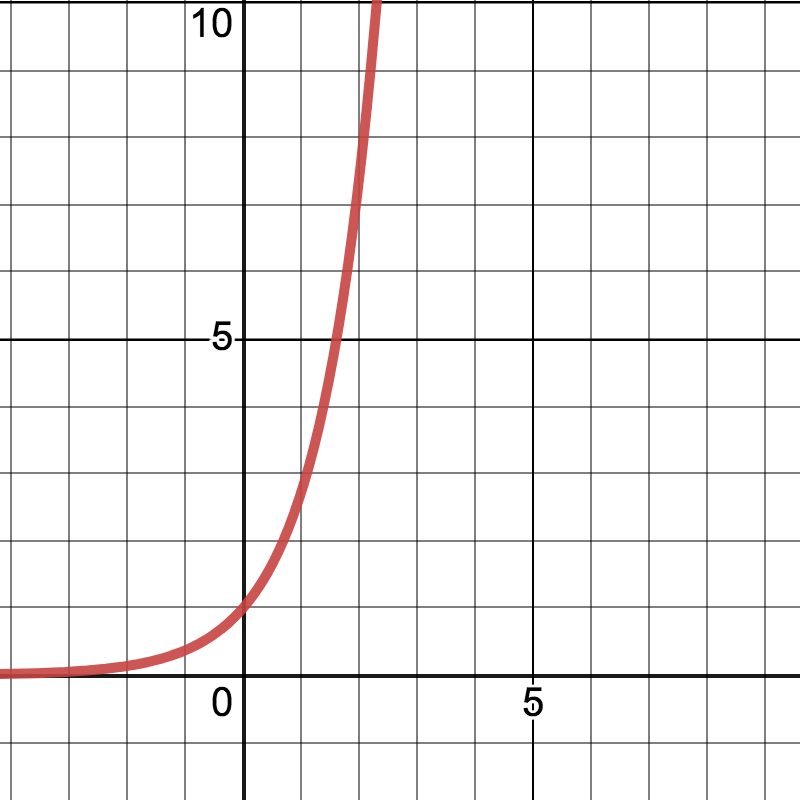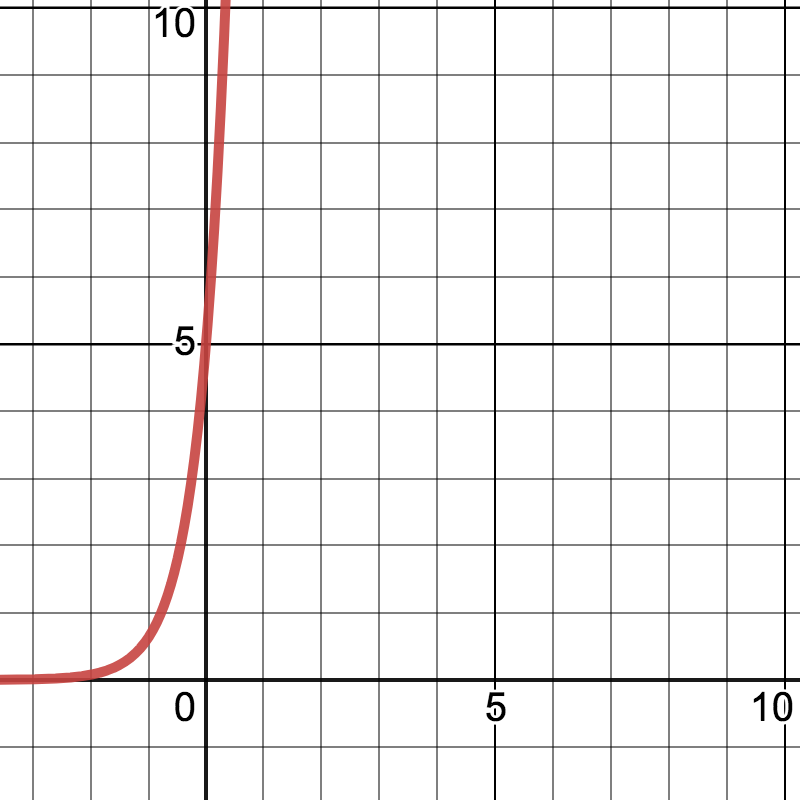### Compound Interest

A special case involving exponential expressions is compound interest. What is compound interest? Usually, the context is of money earning interest in a bank account.

Here is the compound interest formula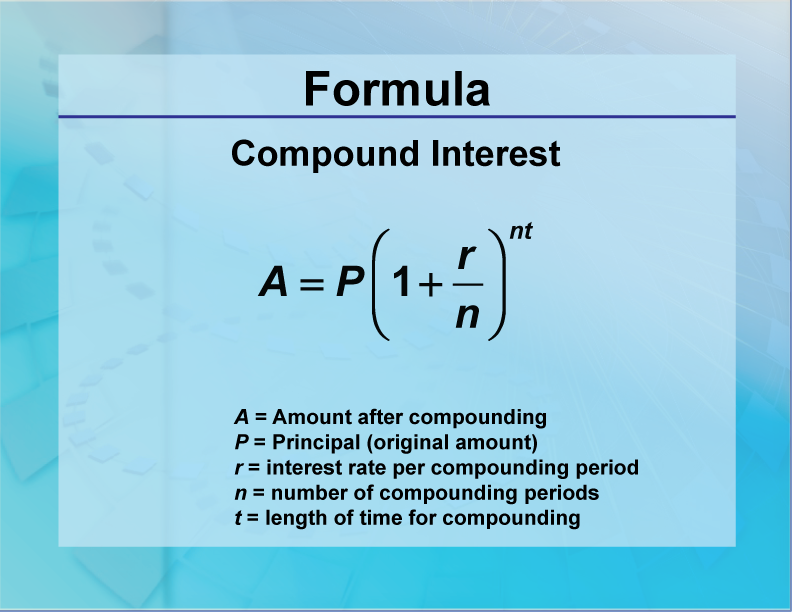This is the formula to use when the compounding periods are non-continuous. Use this formula for continuous compounding: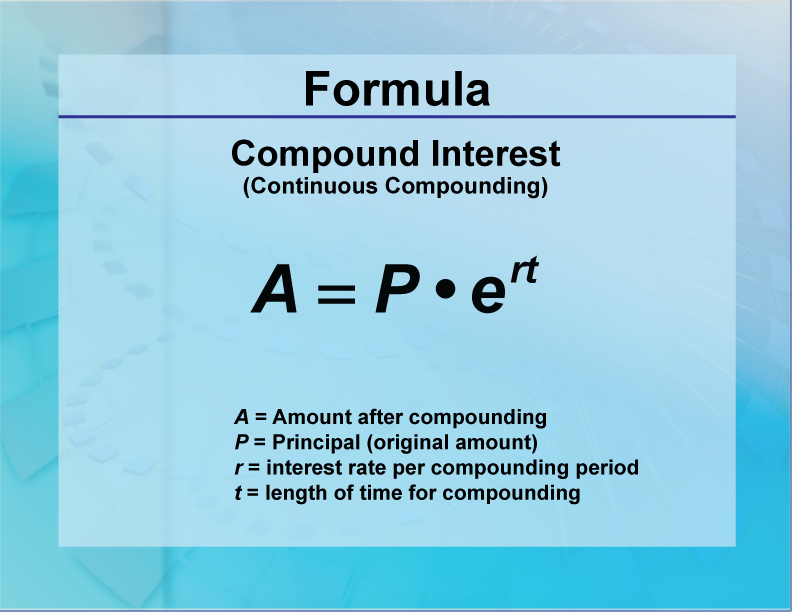To see examples of calculating compound interest, click on this link

 Compound Interest Example 1 A savings account earns interest according the formula below. What is the exponential function that models this growth? How much is in the savings account after five years? “Each year the amount of the account increases by 2.5%. The initial investment was \$1750.” Use the compound interest placeholder formula:In this formula: A = Current amount in savings P = Initial investment r = Interest rate n = Number of compounding periods t = Number of years We know the following: P = 1750 r = 0.025 n = 1 We can now generate the exponential function that models the growth:We can use this model to find the amount in savings after 5 years:## Video Transcripts

### This video has a transcript available. To see the complete collection of video transcripts, click on this link.

Common Core Standards CCSS.MATH.CONTENT.6.SP.B.5, CCSS.MATH.CONTENT.8.SP.A.1, CCSS.MATH.CONTENT.HSS.CP.A.1, CCSS.MATH.CONTENT.HSS.CP.A.2, CCSS.MATH.CONTENT.HSS.CP.A.3, CCSS.MATH.CONTENT.HSS.CP.A.4, CCSS.MATH.CONTENT.HSS.CP.A.5, CCSS.MATH.CONTENT.HSS.CP.B.6, CCSS.MATH.CONTENT.HSS.CP.B.7, CCSS.MATH.CONTENT.HSS.CP.B.8, CCSS.MATH.CONTENT.HSS.CP.B.9 33 minutes 6 - 9 Algebra     • Probability and Data Analysis         • Data Analysis         • Data Gathering 2020 algebra, data analysis, probability, statistics, Closed Captioned Video